The Coterminal Positive Angles Labeled ClipArt gallery offers 73 examples two angles that have the same terminal (or terminating) side. They can be positive or negative integers. This collection shows a positive angle and its negative coterminal angle, and only the positive angles are labeled.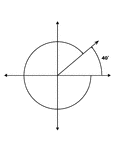### 40° and -320° Coterminal Angles

Illustration showing coterminal angles of 40° and -320°. Coterminal angles are angles drawn…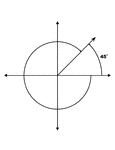### 45° and -315° Coterminal Angles

Illustration showing coterminal angles of 45° and -315°. Coterminal angles are angles drawn…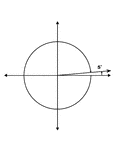### 5° and -355° Coterminal Angles

Illustration showing coterminal angles of 5° and -355°. Coterminal angles are angles drawn in…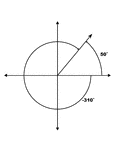### 50° and -310° Coterminal Angles

Illustration showing coterminal angles of 50° and -310°. Coterminal angles are angles drawn…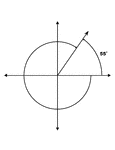### 55° and -305° Coterminal Angles

Illustration showing coterminal angles of 55° and -305°. Coterminal angles are angles drawn…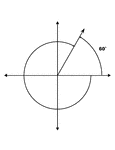### 60° and -300° Coterminal Angles

Illustration showing coterminal angles of 60° and -300°. Coterminal angles are angles drawn…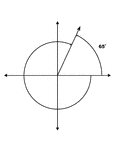### 65° and -295° Coterminal Angles

Illustration showing coterminal angles of 65° and -295°. Coterminal angles are angles drawn…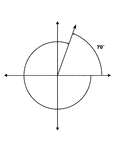### 70° and -290° Coterminal Angles

Illustration showing coterminal angles of 70° and -290°. Coterminal angles are angles drawn…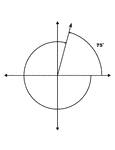### 75° and -285° Coterminal Angles

Illustration showing coterminal angles of 75° and -285°. Coterminal angles are angles drawn…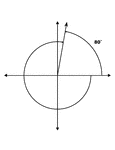### 80° and -280° Coterminal Angles

Illustration showing coterminal angles of 80° and -280°. Coterminal angles are angles drawn…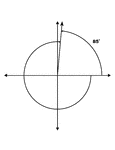### 85° and -275° Coterminal Angles

Illustration showing coterminal angles of 85° and -275°. Coterminal angles are angles drawn…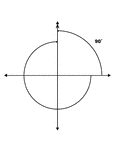### 90° and -270° Coterminal Angles

Illustration showing coterminal angles of 90° and -270°. Coterminal angles are angles drawn…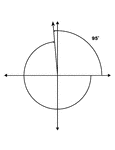### 95° and -265° Coterminal Angles

Illustration showing coterminal angles of 95° and -265°. Coterminal angles are angles drawn…